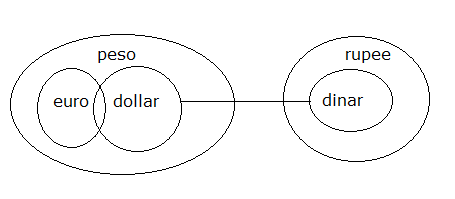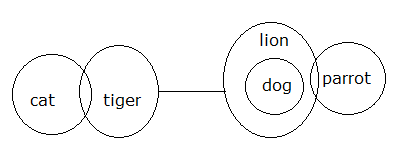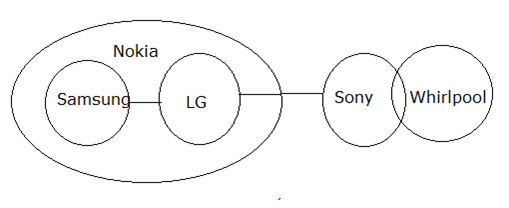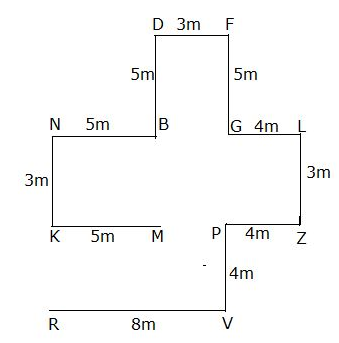# SBI Clerk Pre Reasoning (Day-44)

Dear Aspirants, Our IBPS Guide team is providing new series of Reasoning Questions for SBI Clerk Prelims 2020 so the aspirants can practice it on a daily basis. These questions are framed by our skilled experts after understanding your needs thoroughly. Aspirants can practice these new series questions daily to familiarize with the exact exam pattern and make your preparation effective.

Start Quiz

Ensure Your Ability Before the Exam – Take SBI Clerk 2020 Prelims Free Mock Test

Syllogism:

Directions (1-3): In each of the following questions some statements are given and these statements are followed by two conclusions numbered I and II. You have to take the given two statements to be true even if they seem to be at variance from commonly known facts. Read the conclusions and then decide which of the given conclusions logically follows from the two given statements, disregarding commonly known facts.

Give answer:

(a) If only I conclusion follows

(b) If only II conclusion follows

(c) If either conclusion I or II follows

(d) If neither conclusion I nor II follows and

(e) If both conclusions I and II follow

1) Statements:

Some euro are dollar

No dollar is dinar

All dinar are rupee

All euro are peso

All dollar are peso

Conclusions:

I) Some dollar are rupee

II) Some peso are not rupee

2) Statements:

Some cat are tiger

No tiger is lion

All dog are lion

Some lion are parrot

Conclusions:

I) Some parrot are not cat

II) Some dog are not tiger

3) Statements:

No Samsung is LG

No LG is Sony

Some Sony are whirlpool

All Samsung are Nokia

All LG are Nokia

Conclusions:

I) Some whirlpool are not LG

II) Some Samsung are Sony is a possibility

Inequality:

Directions (4-6): In these questions, relationship between different elements is shown in the statements. The statements are followed by conclusions.

Give Answer

(a) If only I conclusion follows

(b) If only II conclusion follows

(c) If either conclusions I or II follows

(d) If neither conclusions I nor II follows and

(e) If both conclusions I and II follow.

4) Statements:

D ≤ M ≥ K > S ≥ W; V ≥ S ≥ L; D ≤ M ≥ P

Conclusions:

I) P > S

II) D ≥ L

5) Statements:

R ≤ S < M ≤ X; B ≥ Z > R; M > F

Conclusions:

I) B < M

II) X ≥ F

6) Statements:

K ≥ Q ≥ D > G; Q ≤ C ≤ L ; J < T < D

Conclusions:

I) D ≤ L

II) J > L

Direction sense:

Directions (7-8): Study the following information carefully and answer the questions given below:

Point K is 5m west of Point M. Point N is 3m north of Point K. Point B is 5m east of Point N. Point D is 5m north of Point B. Point F is 3m east of Point D. Point G is 5m south of Point F. Point L is 4m east of Point G. Point Z is 3m south of Point L. Point P is 4m west of Point Z. Point V is 4m south of Point P. Point R is 8m west of Point V.

7) How far and in which direction Point M with respect to Point V?

a) 5m, North East

b) 5m, North West

c) 4m, South East

d) 4m, South West

e) None of these

8) Four of the following five are alike in a certain way based on the group. Find the one which does not belongs to the group?

a) MR

b) FB

c) LP

d) GM

e) DG

Order and ranking:

Directions (9-10): Study the following information carefully and answer the questions given below:

Six cities L, B, G, R, K and F have different lakes.  City B has more number of lakes than city G but have less number of lakes than city F. City F does not have the maximum number of lakes. Only one city has less number of lakes than city K. City L does not have least number of lakes. G does not has least number of lakes.

9) Which city has maximum number of lakes?

a) F

b) B

c) L

d) G

e) None of these

10) How many cities have less number of lakes than city B?

a) 1

b) None

c) 2

d) 3

e) None of these

Answers :

Directions (1-3):

1) Answer: (d)2) Answer: (b)3) Answer: (e)Directions (4-6):

4) Answer: (d)

I) P > S (P ≤ M ≥ K > S)

II) D ≥ L (D ≤ M ≥ K > S ≥ L)

5) Answer:  (d)

I) B < M (B ≥ Z > R ≤ S < M)

II) X ≥ F (F < M ≤  X)

6) Answer: (a)

I) D ≤ L (L ≥ C ≥ Q ≥ D)

II) J > L (J < T < D > L)

Directions (7-8):7) Answer: (b)

8) Answer: (e)

Directions (9-10):

L > F > B > G > K > R

9) Answer: (c)

10) Answer:  (d)

 Check Here to View SBI Clerk Prelims 2020 Reasoning Ability Questions Day – 43 Day – 42 Day – 41 Click Here for SBI Clerk 2020 – Detailed Exam Notification
0 0 votes
Rating0 Comments
Inline Feedbacks
View all comments